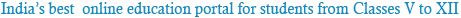### NCERT Solutions for Class 10th Science: Chapter 12: Electricity

Question 1:
What does an electric circuit mean?
An electric circuit consists of electric devices, switching devices, source of electricity, etc. that are connected by conducting wires.

Question 2:
Define the unit of current.
The unit of electric current is ampere (A). 1 A is defined as the flow of 1 C of charge through a wire in 1 s.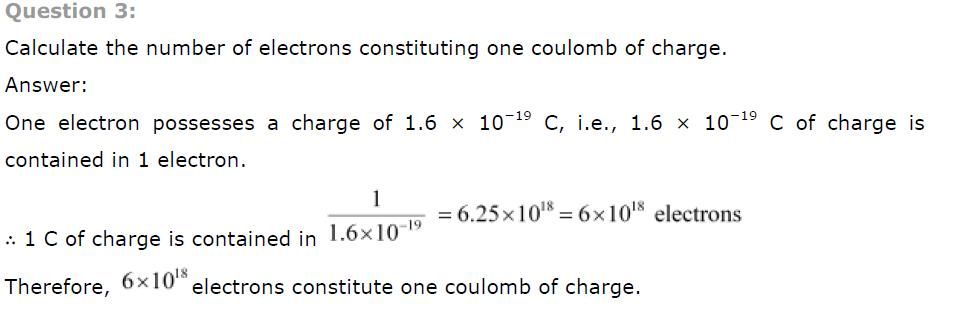Question 1:
Name a device that helps to maintain a potential difference across a conductor.
A source of electricity such as cell, battery, power supply, etc. helps to maintain a potential difference across a conductor.

Question 2:
What is meant by saying that the potential difference between two points is 1 V?
If 1 J of work is required to move a charge of amount 1 C from one point to another, then it is said that the potential difference between the two points is 1 V.Question 1:
On what factors does the resistance of a conductor depend?
The resistance of a conductor depends upon the following factors:
(a) Length of the conductor
(b) Cross-sectional area of the conductor
(c) Material of the conductor
(d) Temperature of the conductorPotential difference = V
Current = I
The potential difference is reduced to half, keeping resistance constant.
Let the new resistance be R’ and the new amount of current be I ‘.
Therefore, from Ohm’s law, we obtain the amount of new current.Therefore, the amount of current flowing through the electrical component is reduced by half.
Question 4:
Why are coils of electric toasters and electric irons made of an alloy rather than a pure metal?
The resistivity of an alloy is higher than the pure metal. Moreover, at high temperatures, the alloys do not melt readily. Hence, the coils of heating appliances such as electric toasters and electric irons are made of an alloy rather than a pure metal.
Question 5:
Use the data in Table 12.2 to answer the following −
Table 12.2 Electrical resistivity of some substances at 20°CQuestion 1:
Draw a schematic diagram of a circuit consisting of a battery of three cells of 2 V each, a 5 G resistor, an 8 G resistor, and a 12 G resistor, and a plug key, all connected in series.
Three cells of potential 2 V, each connected in series, is equivalent to a battery of potential 2 V + 2 V + 2 V = 6V. The following circuit diagram shows three resistors of resistances 5 G, 8 G and 12 G respectively connected in series and a battery of potential 6 V.Question 2:
Redraw the circuit of question 1, putting in an ammeter to measure the current through the resistors and a voltmeter to measure potential difference across the 12 G resistor.
What would be the readings in the ammeter and the voltmeter?
To measure the current flowing through the resistors, an ammeter should be connected in the circuit in series with the resistors. To measure the potential difference across the 12 G resistor, a voltmeter should be connected parallel to this resistor, as shown in the following figure.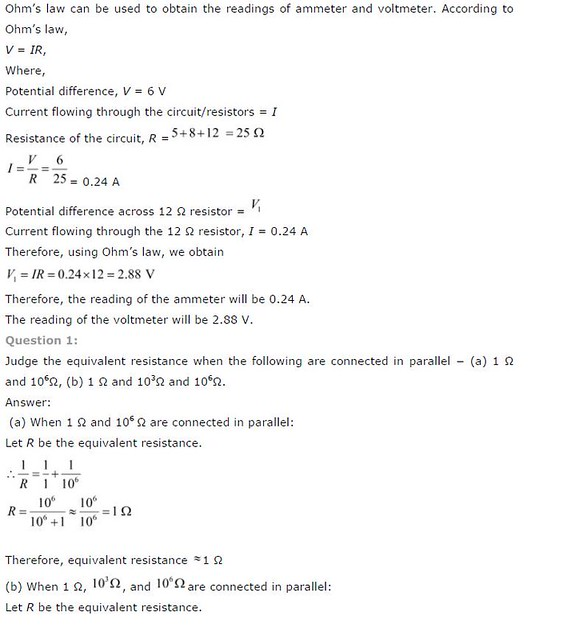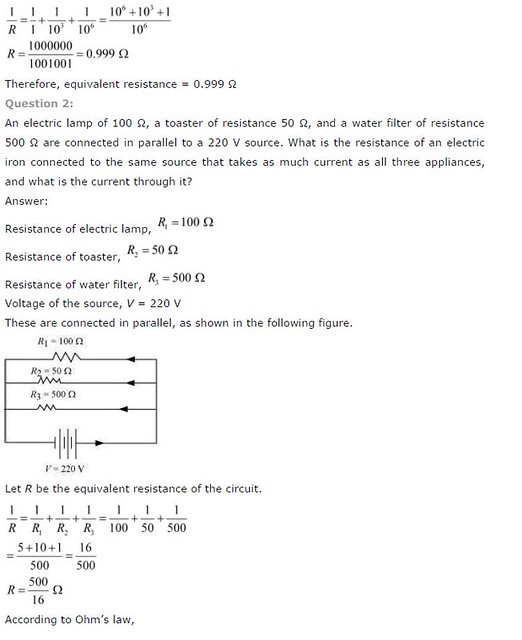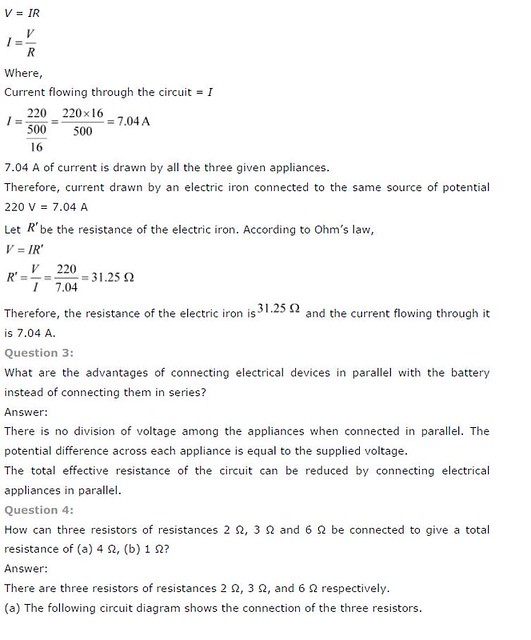Question 1:
Why does the cord of an electric heater not glow while the heating element does?
The heating element of an electric heater is a resistor. The amount of heat produced by it is proportional to its resistance. The resistance of the element of an electric heater is very high. As current flows through the heating element, it becomes too hot and glows red. On the other hand, the resistance of the cord is low. It does not become red when current flows through it.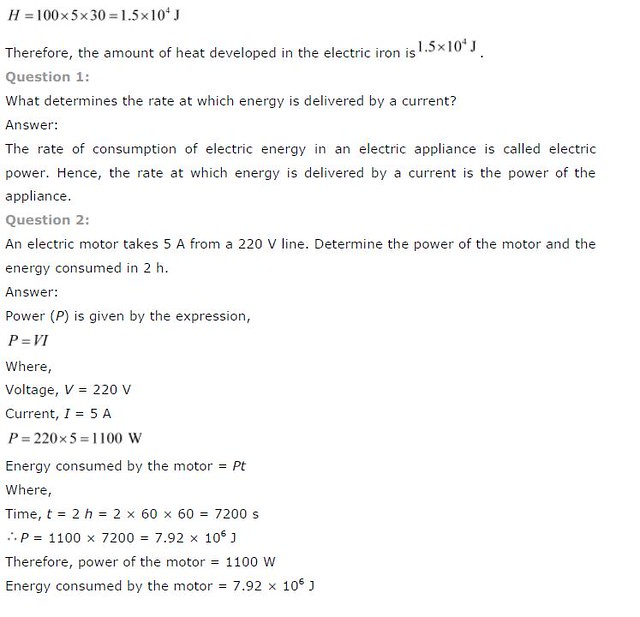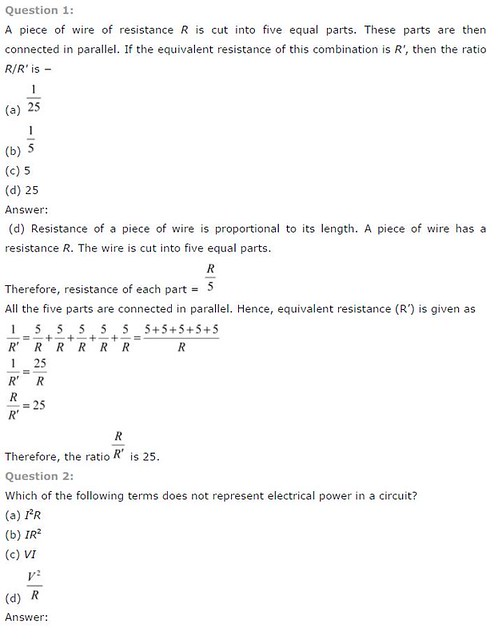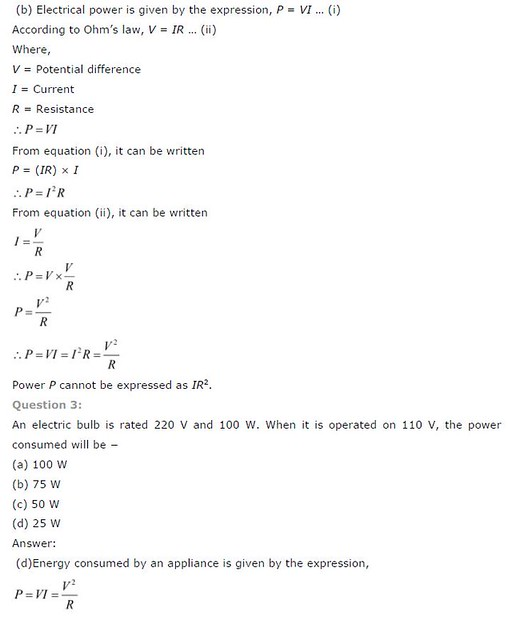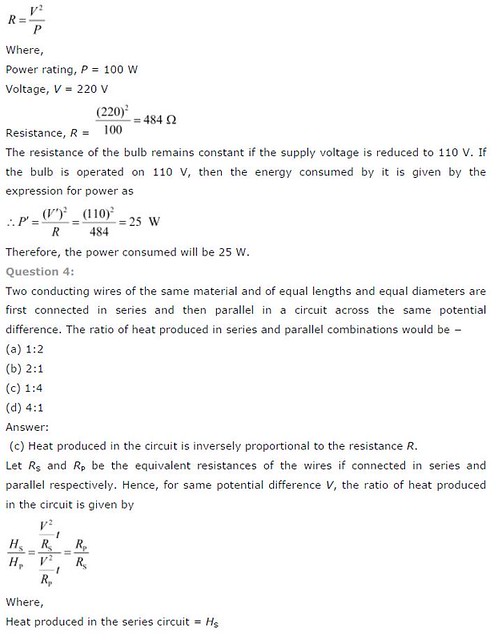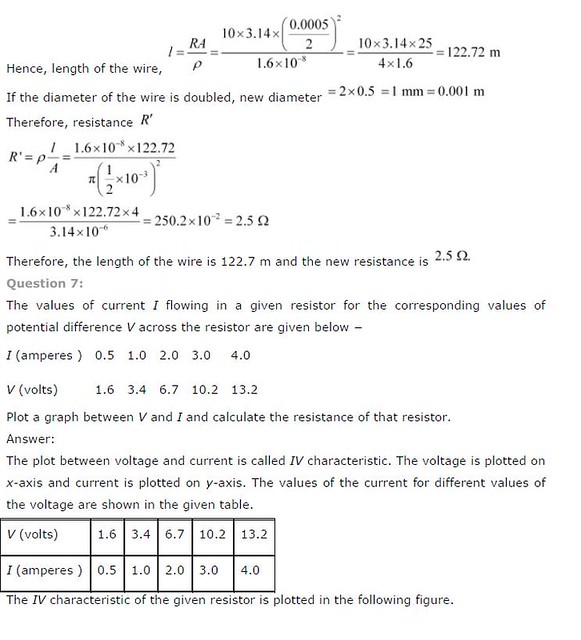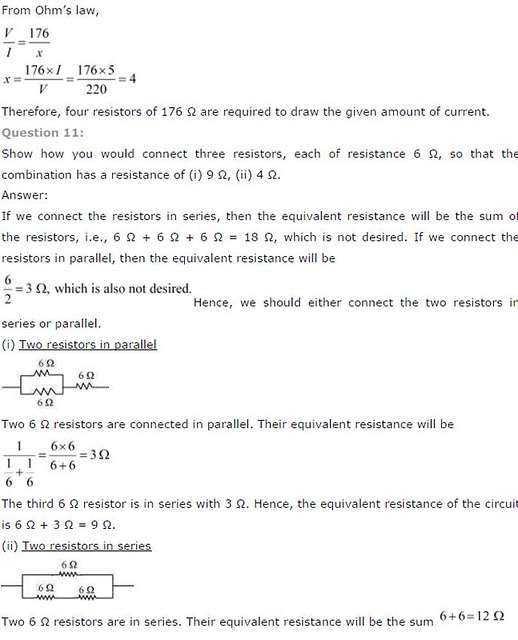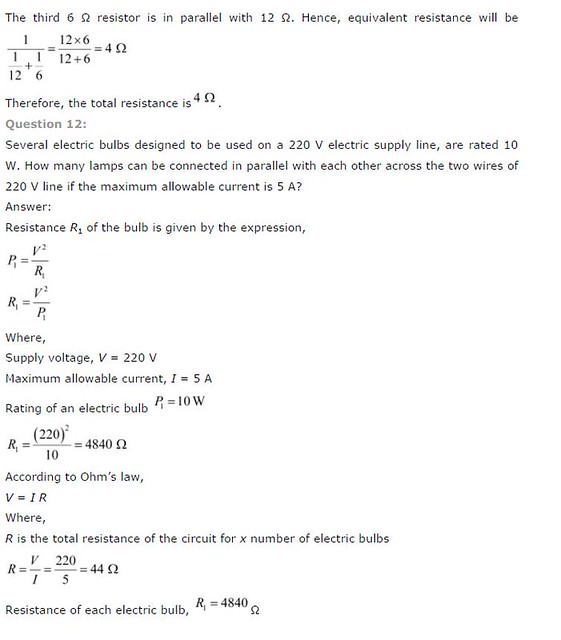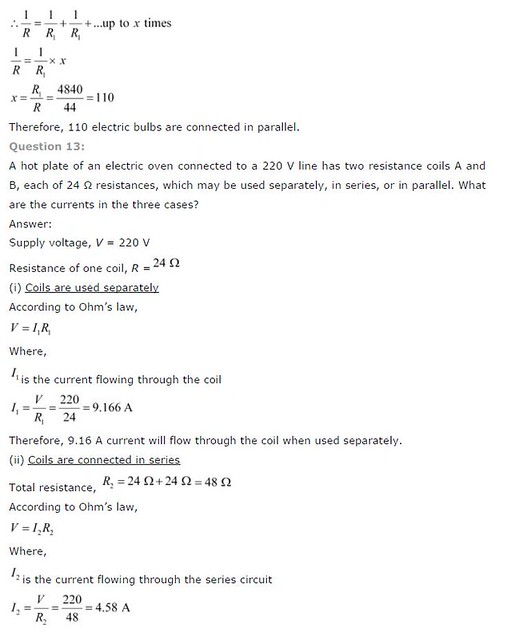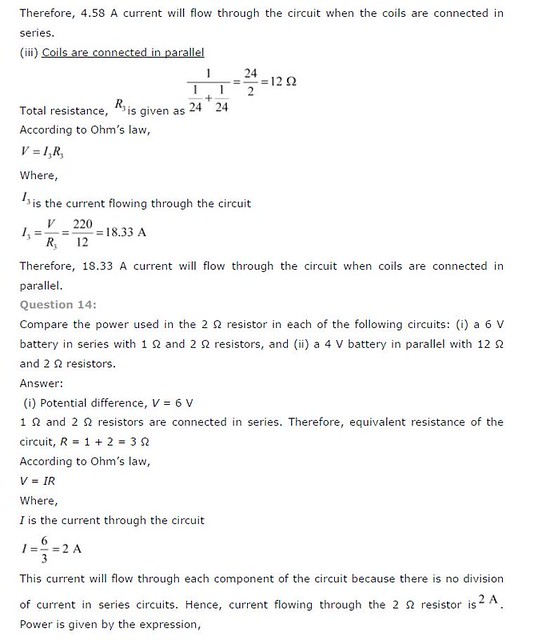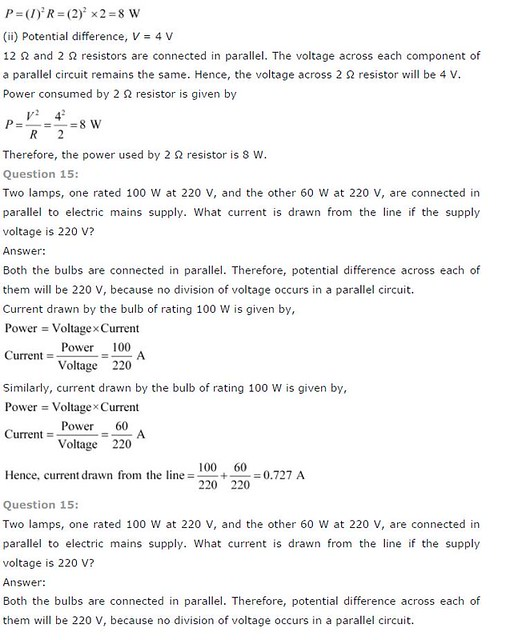Powered by: iVerticals Web Technology Pvt Ltd